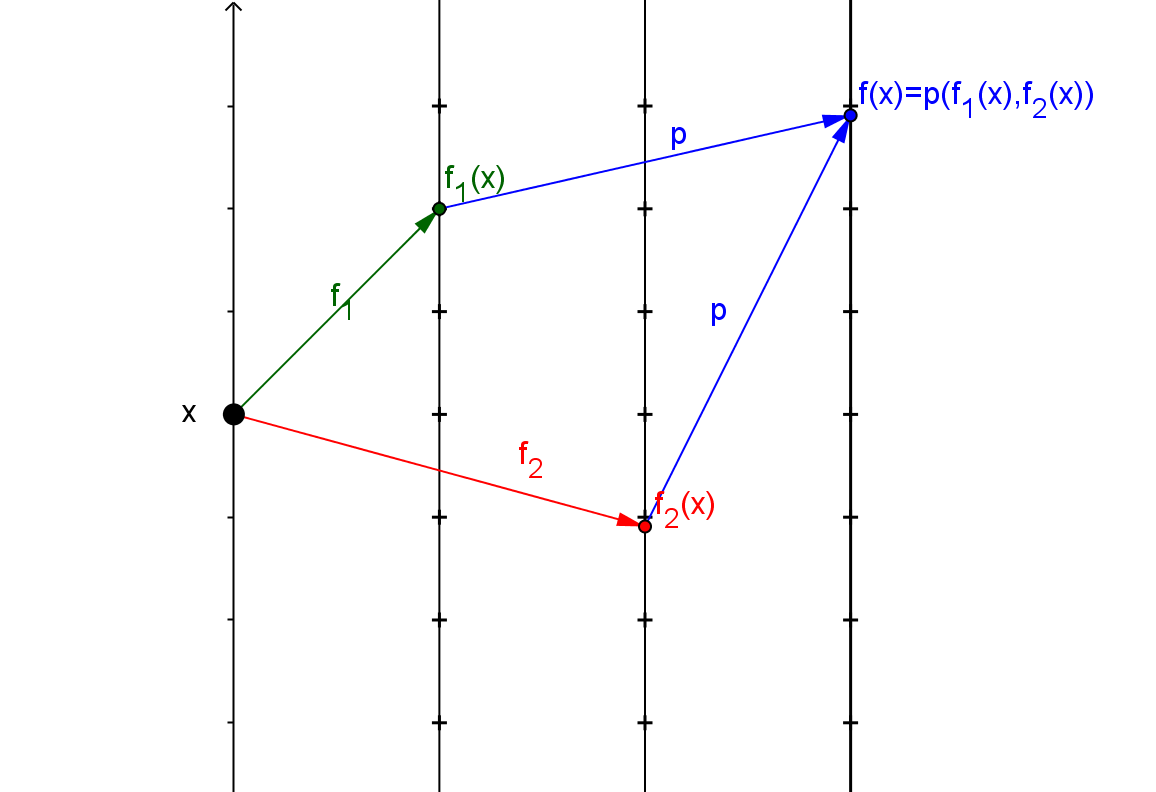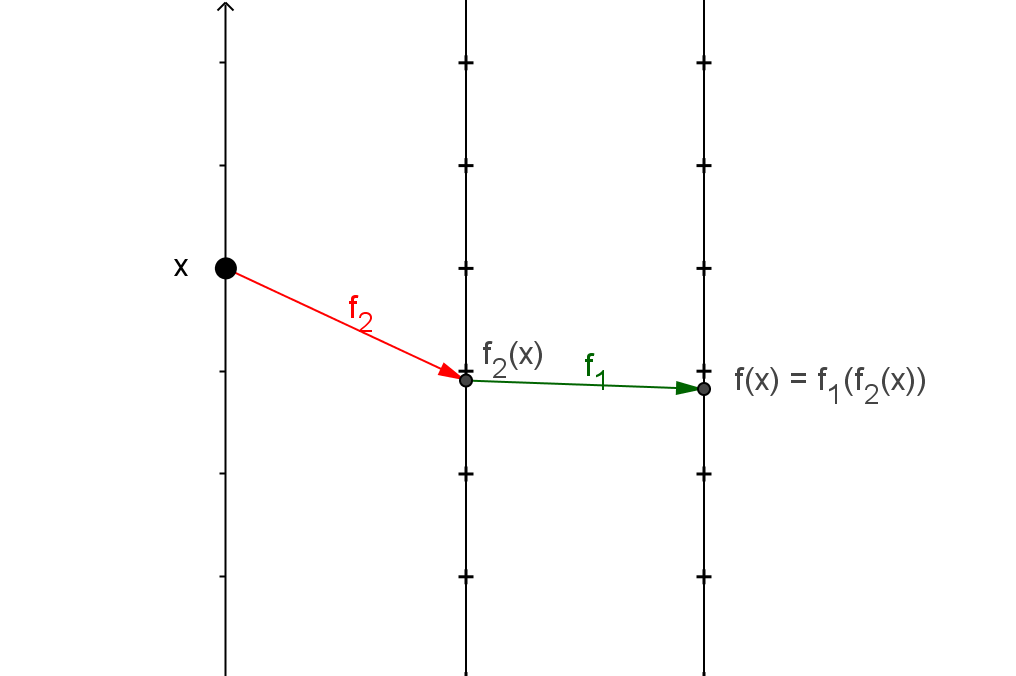Suppose $f$ is a real valued function with domain a subset of  real numbers, $D$.
Definition: $f$  is an core function if $f \in \{f_k, id, \exp, \sin\}$
where $f_k(x) = k$ for all $x$, $id(x)=x$ for all $x$, $\exp(x) = e^x$ for all $x$, and $\sin(x)$ is the trigonometric function, sine.
Alternative Definition: $f$  is an core function if $f \in \{f_k, id, x^n,\exp, \ln, \sin, \cos, \tan \}$
Definition:
$f$  is an elementary function if
(i)$f$ is a core function
or(ii) there are elementary functions $f_1$ and $f_2$ so that for any $x \in D$,
either $f(x) = p(f_1(x),f_2(x))$ where $p(a,b) \in \{ a+b, a-b, a*b, a/b\}$
or $f(x) = f_1(f_2(x))$or

(iii) there is an elementary function, $g$, where $f$ is the inverse function for $g$,  i.e. $f (g(x)) =x$ and $g(f(x))= x$ for suitable values of $x$.